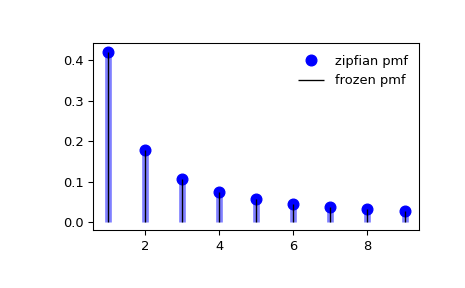# scipy.stats.zipfian¶

scipy.stats.zipfian = <scipy.stats._discrete_distns.zipfian_gen object>[source]

A Zipfian discrete random variable.

As an instance of the rv_discrete class, zipfian object inherits from it a collection of generic methods (see below for the full list), and completes them with details specific for this particular distribution.

Notes

The probability mass function for zipfian is:

$f(k, a, n) = \frac{1}{H_{n,a} k^a}$

for $$k \in \{1, 2, \dots, n-1, n\}$$, $$a \ge 0$$, $$n \in \{1, 2, 3, \dots\}$$.

zipfian takes $$a$$ and $$n$$ as shape parameters. $$H_{n,a}$$ is the $$n$$th generalized harmonic number of order $$a$$.

The Zipfian distribution reduces to the Zipf (zeta) distribution as $$n \rightarrow \infty$$.

The probability mass function above is defined in the “standardized” form. To shift distribution use the loc parameter. Specifically, zipfian.pmf(k, a, n, loc) is identically equivalent to zipfian.pmf(k - loc, a, n).

References

1

“Zipf’s Law”, Wikipedia, https://en.wikipedia.org/wiki/Zipf’s_law

2

Larry Leemis, “Zipf Distribution”, Univariate Distribution Relationships. http://www.math.wm.edu/~leemis/chart/UDR/PDFs/Zipf.pdf

Examples

>>> from scipy.stats import zipfian
>>> import matplotlib.pyplot as plt
>>> fig, ax = plt.subplots(1, 1)


Calculate the first four moments:

>>> a, n = 1.25, 10
>>> mean, var, skew, kurt = zipfian.stats(a, n, moments='mvsk')


Display the probability mass function (pmf):

>>> x = np.arange(zipfian.ppf(0.01, a, n),
...               zipfian.ppf(0.99, a, n))
>>> ax.plot(x, zipfian.pmf(x, a, n), 'bo', ms=8, label='zipfian pmf')
>>> ax.vlines(x, 0, zipfian.pmf(x, a, n), colors='b', lw=5, alpha=0.5)


Alternatively, the distribution object can be called (as a function) to fix the shape and location. This returns a “frozen” RV object holding the given parameters fixed.

Freeze the distribution and display the frozen pmf:

>>> rv = zipfian(a, n)
>>> ax.vlines(x, 0, rv.pmf(x), colors='k', linestyles='-', lw=1,
...         label='frozen pmf')
>>> ax.legend(loc='best', frameon=False)
>>> plt.show()Check accuracy of cdf and ppf:

>>> prob = zipfian.cdf(x, a, n)
>>> np.allclose(x, zipfian.ppf(prob, a, n))
True


Generate random numbers:

>>> r = zipfian.rvs(a, n, size=1000)


Confirm that zipfian reduces to zipf for large n, a > 1.

>>> from scipy.stats import zipf
>>> k = np.arange(11)
>>> np.allclose(zipfian.pmf(k, a=3.5, n=10000000), zipf.pmf(k, a=3.5))
True


Methods

 rvs(a, n, loc=0, size=1, random_state=None) Random variates. pmf(k, a, n, loc=0) Probability mass function. logpmf(k, a, n, loc=0) Log of the probability mass function. cdf(k, a, n, loc=0) Cumulative distribution function. logcdf(k, a, n, loc=0) Log of the cumulative distribution function. sf(k, a, n, loc=0) Survival function (also defined as 1 - cdf, but sf is sometimes more accurate). logsf(k, a, n, loc=0) Log of the survival function. ppf(q, a, n, loc=0) Percent point function (inverse of cdf — percentiles). isf(q, a, n, loc=0) Inverse survival function (inverse of sf). stats(a, n, loc=0, moments=’mv’) Mean(‘m’), variance(‘v’), skew(‘s’), and/or kurtosis(‘k’). entropy(a, n, loc=0) (Differential) entropy of the RV. expect(func, args=(a, n), loc=0, lb=None, ub=None, conditional=False) Expected value of a function (of one argument) with respect to the distribution. median(a, n, loc=0) Median of the distribution. mean(a, n, loc=0) Mean of the distribution. var(a, n, loc=0) Variance of the distribution. std(a, n, loc=0) Standard deviation of the distribution. interval(alpha, a, n, loc=0) Endpoints of the range that contains fraction alpha [0, 1] of the distribution